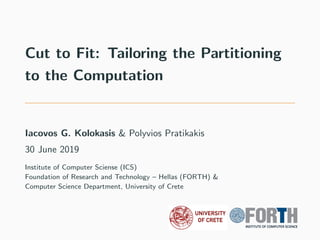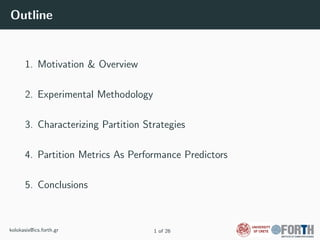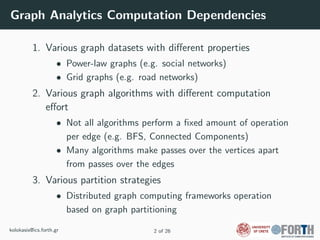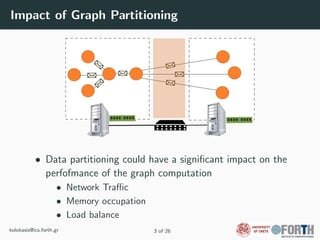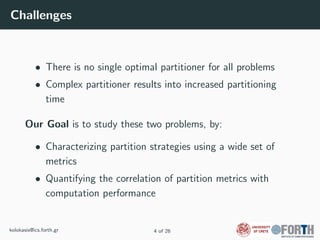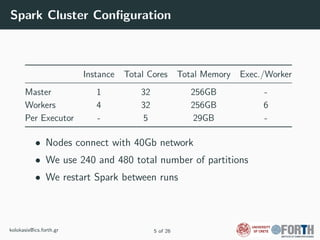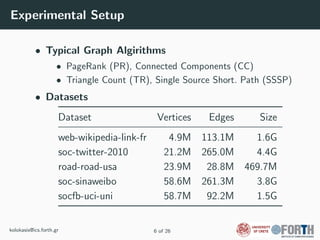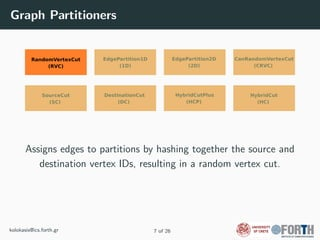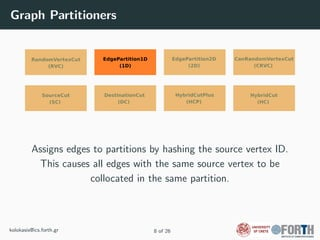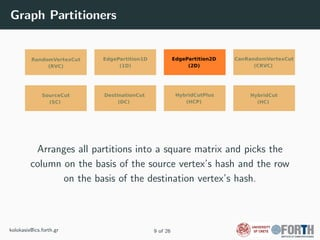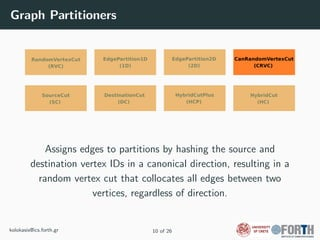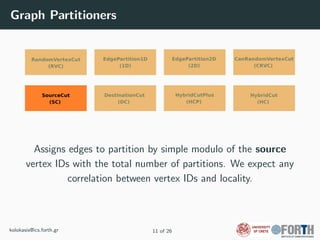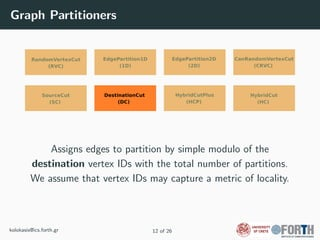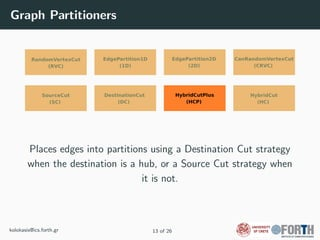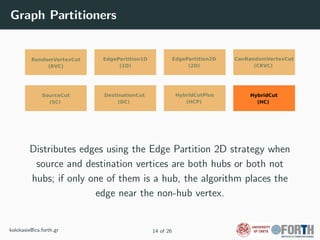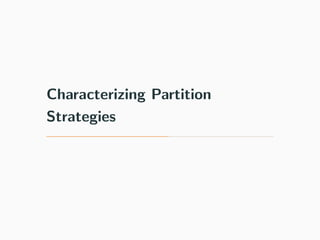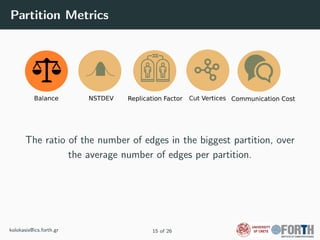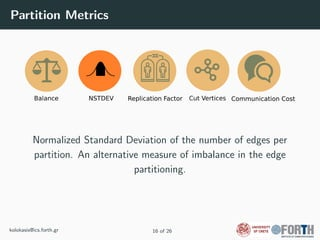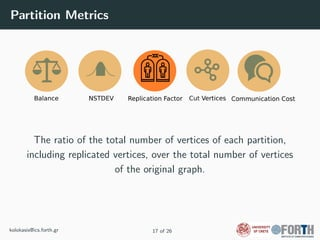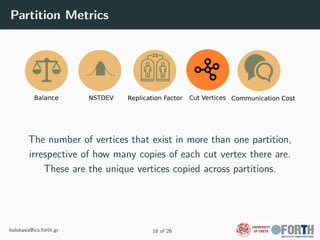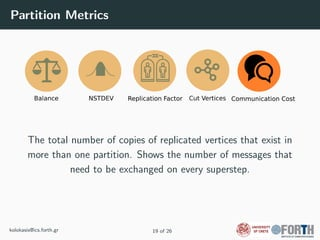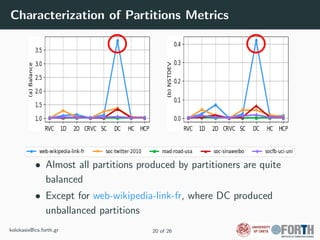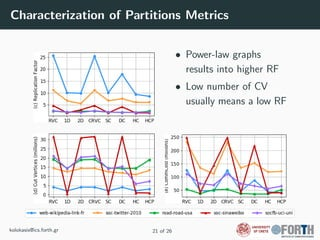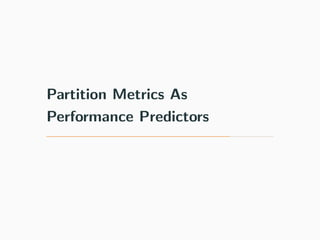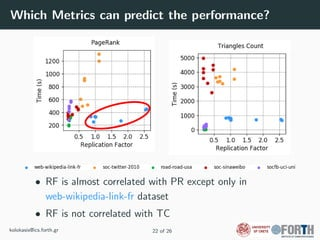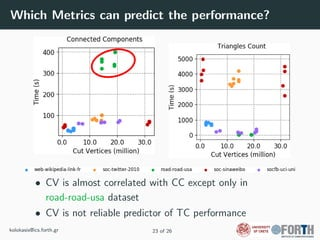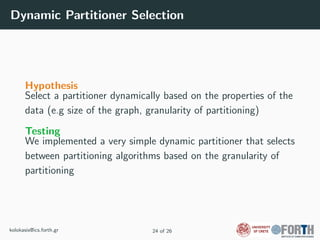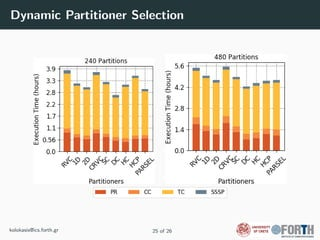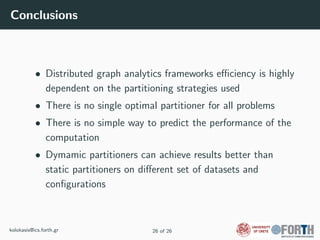1 of 33

### Cut to Fit: Tailoring the Partitioning to the Computation

1. Cut to Fit: Tailoring the Partitioning to the Computation Iacovos G. Kolokasis & Polyvios Pratikakis 30 June 2019 Institute of Computer Sciense (ICS) Foundation of Research and Technology – Hellas (FORTH) & Computer Science Department, University of Crete
2. Outline 1. Motivation & Overview 2. Experimental Methodology 3. Characterizing Partition Strategies 4. Partition Metrics As Performance Predictors 5. Conclusions kolokasis@ics.forth.gr 1 of 26
3. Motivation & Overview
4. Graph Analytics Computation Dependencies 1. Various graph datasets with diﬀerent properties • Power-law graphs (e.g. social networks) • Grid graphs (e.g. road networks) 2. Various graph algorithms with diﬀerent computation eﬀort • Not all algorithms perform a ﬁxed amount of operation per edge (e.g. BFS, Connected Components) • Many algorithms make passes over the vertices apart from passes over the edges 3. Various partition strategies • Distributed graph computing frameworks operation based on graph partitioning kolokasis@ics.forth.gr 2 of 26
5. Impact of Graph Partitioning • Data partitioning could have a signiﬁcant impact on the perfofmance of the graph computation • Network Traﬃc • Memory occupation • Load balance kolokasis@ics.forth.gr 3 of 26
6. Challenges • There is no single optimal partitioner for all problems • Complex partitioner results into increased partitioning time Our Goal is to study these two problems, by: • Characterizing partition strategies using a wide set of metrics • Quantifying the correlation of partition metrics with computation performance kolokasis@ics.forth.gr 4 of 26
7. Experimental Methodology
8. Spark Cluster Conﬁguration Instance Total Cores Total Memory Exec./Worker Master 1 32 256GB - Workers 4 32 256GB 6 Per Executor - 5 29GB - • Nodes connect with 40Gb network • We use 240 and 480 total number of partitions • We restart Spark between runs kolokasis@ics.forth.gr 5 of 26
9. Experimental Setup • Typical Graph Algirithms • PageRank (PR), Connected Components (CC) • Triangle Count (TR), Single Source Short. Path (SSSP) • Datasets Dataset Vertices Edges Size web-wikipedia-link-fr 4.9M 113.1M 1.6G soc-twitter-2010 21.2M 265.0M 4.4G road-road-usa 23.9M 28.8M 469.7M soc-sinaweibo 58.6M 261.3M 3.8G socfb-uci-uni 58.7M 92.2M 1.5G kolokasis@ics.forth.gr 6 of 26
10. Graph Partitioners Assigns edges to partitions by hashing together the source and destination vertex IDs, resulting in a random vertex cut. kolokasis@ics.forth.gr 7 of 26
11. Graph Partitioners Assigns edges to partitions by hashing the source vertex ID. This causes all edges with the same source vertex to be collocated in the same partition. kolokasis@ics.forth.gr 8 of 26
12. Graph Partitioners Arranges all partitions into a square matrix and picks the column on the basis of the source vertex’s hash and the row on the basis of the destination vertex’s hash. kolokasis@ics.forth.gr 9 of 26
13. Graph Partitioners Assigns edges to partitions by hashing the source and destination vertex IDs in a canonical direction, resulting in a random vertex cut that collocates all edges between two vertices, regardless of direction. kolokasis@ics.forth.gr 10 of 26
14. Graph Partitioners Assigns edges to partition by simple modulo of the source vertex IDs with the total number of partitions. We expect any correlation between vertex IDs and locality. kolokasis@ics.forth.gr 11 of 26
15. Graph Partitioners Assigns edges to partition by simple modulo of the destination vertex IDs with the total number of partitions. We assume that vertex IDs may capture a metric of locality. kolokasis@ics.forth.gr 12 of 26
16. Graph Partitioners Places edges into partitions using a Destination Cut strategy when the destination is a hub, or a Source Cut strategy when it is not. kolokasis@ics.forth.gr 13 of 26
17. Graph Partitioners Distributes edges using the Edge Partition 2D strategy when source and destination vertices are both hubs or both not hubs; if only one of them is a hub, the algorithm places the edge near the non-hub vertex. kolokasis@ics.forth.gr 14 of 26
18. Characterizing Partition Strategies
19. Partition Metrics The ratio of the number of edges in the biggest partition, over the average number of edges per partition. kolokasis@ics.forth.gr 15 of 26
20. Partition Metrics Normalized Standard Deviation of the number of edges per partition. An alternative measure of imbalance in the edge partitioning. kolokasis@ics.forth.gr 16 of 26
21. Partition Metrics The ratio of the total number of vertices of each partition, including replicated vertices, over the total number of vertices of the original graph. kolokasis@ics.forth.gr 17 of 26
22. Partition Metrics The number of vertices that exist in more than one partition, irrespective of how many copies of each cut vertex there are. These are the unique vertices copied across partitions. kolokasis@ics.forth.gr 18 of 26
23. Partition Metrics The total number of copies of replicated vertices that exist in more than one partition. Shows the number of messages that need to be exchanged on every superstep. kolokasis@ics.forth.gr 19 of 26
24. Characterization of Partitions Metrics • Almost all partitions produced by partitioners are quite balanced • Except for web-wikipedia-link-fr, where DC produced unballanced partitions kolokasis@ics.forth.gr 20 of 26
25. Characterization of Partitions Metrics • Power-law graphs results into higher RF • Low number of CV usually means a low RF kolokasis@ics.forth.gr 21 of 26
26. Partition Metrics As Performance Predictors
27. Which Metrics can predict the performance? • RF is almost correlated with PR except only in web-wikipedia-link-fr dataset • RF is not correlated with TC kolokasis@ics.forth.gr 22 of 26
28. Which Metrics can predict the performance? • CV is almost correlated with CC except only in road-road-usa dataset • CV is not reliable predictor of TC performance kolokasis@ics.forth.gr 23 of 26
29. Dynamic Partitioner Selection Hypothesis Select a partitioner dynamically based on the properties of the data (e.g size of the graph, granularity of partitioning) Testing We implemented a very simple dynamic partitioner that selects between partitioning algorithms based on the granularity of partitioning kolokasis@ics.forth.gr 24 of 26
30. Dynamic Partitioner Selection kolokasis@ics.forth.gr 25 of 26
31. Conclusions
32. Conclusions • Distributed graph analytics frameworks eﬃciency is highly dependent on the partitioning strategies used • There is no single optimal partitioner for all problems • There is no simple way to predict the performance of the computation • Dymamic partitioners can achieve results better than static partitioners on diﬀerent set of datasets and conﬁgurations kolokasis@ics.forth.gr 26 of 26
33. Q&A For questions after this session, contact us at: kolokasis@ics.forth.gr Supported by: